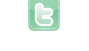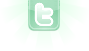Report as inappropriate.

# Lab 4 – Parallax scrolling

No description.

Your browser does not support the canvas tag.
This is really great job, it's awesome, thanks for putting the source I have one question, does anybody know how can I use my own star images? instead of adding object from greenfoot? I know the method but I don't know how to put it in the code, I mean i can use setImage but should i remove all statements in if ??? can u plzzz help me ...
if you want the stars to look different you just have to change the constructor of the star class. (public Star(int speed, ...)) Instead of the image that is used there you can use your own image
but this will change the stars for only one layer, I mean the stars will be the same for all three layers, while what i want is different stars for different layers
you can also change the stars into different images using a random or the value for the speed of them.
Dear Malmötri, I just wanna give u a random number of thanks for ur post with code opening!! But let me understand something more details of a part of your program in the "Star" class I'm gonna mention down: public Star(int speed, Color color) { radius = 1 + Greenfoot.getRandomNumber(4); GreenfootImage image = new GreenfootImage (radius, radius); image.setColor (color); image.fillOval (0, 0, radius, radius); setImage (image); this.speed=speed; x = Greenfoot.getRandomNumber(800); y = Greenfoot.getRandomNumber(600); } public void move() { x = speed + x; setLocation(x + speed, y); if(x<1){ x=600;} } } Questions: 1. Why do we need radius and what is actually happening in the line "radius = 1 + Greenfoot.getRandomNumber(4);"? 2. What is the perpose of the codes "{ x = speed + x; setLocation(x + speed, y); if(x<1){ x=600;} } "?? 3. And tell me a little bit more about these two lines: " x = Greenfoot.getRandomNumber(800); y = Greenfoot.getRandomNumber(600);"
@hkrhassleholm, (1) radius is actually the size of the star (in pixels). It is not really being used as a radius, but as a diameter here. The next line creates an image with that size and two lines later the circle (oval) drawn on the image also uses it as the diameter of the star. (2) this is the part of the code that moves the star. For some reason (or for no reason) this code is adding speed to the x location twice; once in the 'x =...' statement and once in the 'setlocation(...' statement. That just makes each star move twice its speed. The last statement will 'wrap' the star around the world: if on left edge, set the next x to at the right edge. (3) x and y, here, will be used to place a star in the world. The 800 (which is the width of the world) and the 600 (which is the height of the world) ensure that what is returned will be somewhere in the world. Whatever the number (800, 600, 2), it is the number of possible returns, starting at zero and ending at one less than that number.
@hkrhassleholm, the 800 and 600 were supposed to be 600 and 400 for the width and height of the world; that is why the move code sets x back to 600.
What is the scrolling code please.
@comandodude101, the scrolling code is the 'act' method of the world class which will only move each star in the world the distance determined by each star's speed value. The 'background' is a flat black color and does not move at all; therefore, this would not be much help.